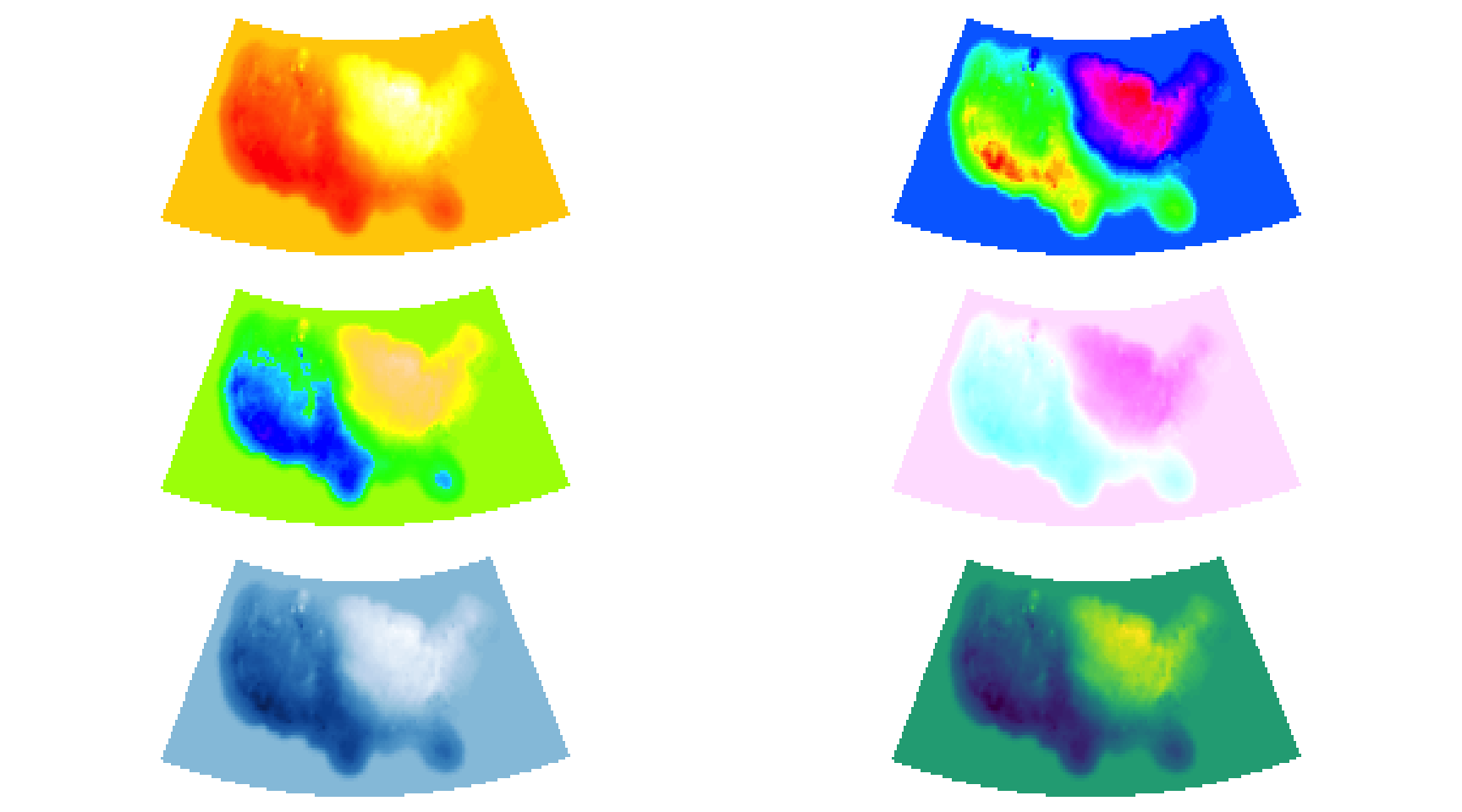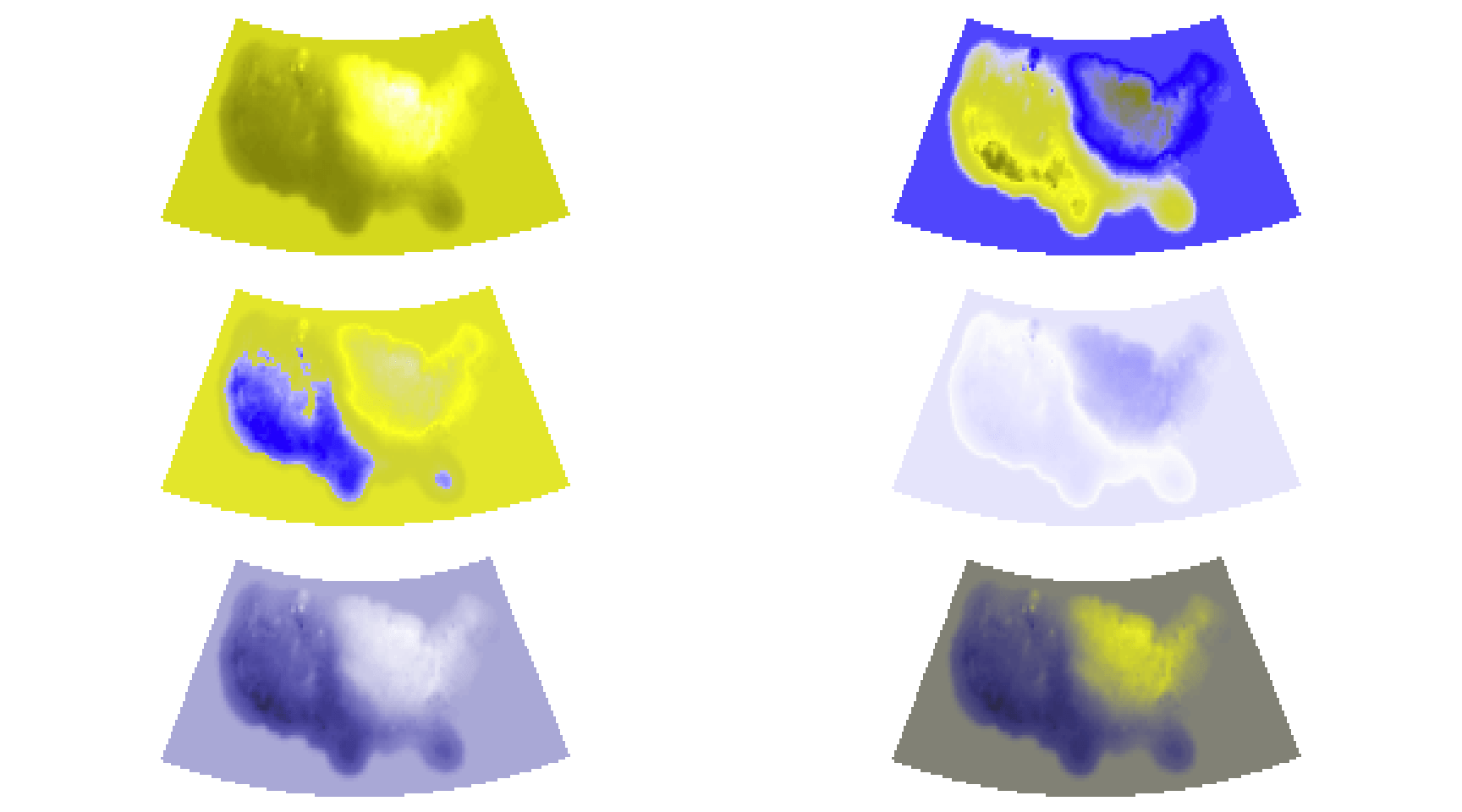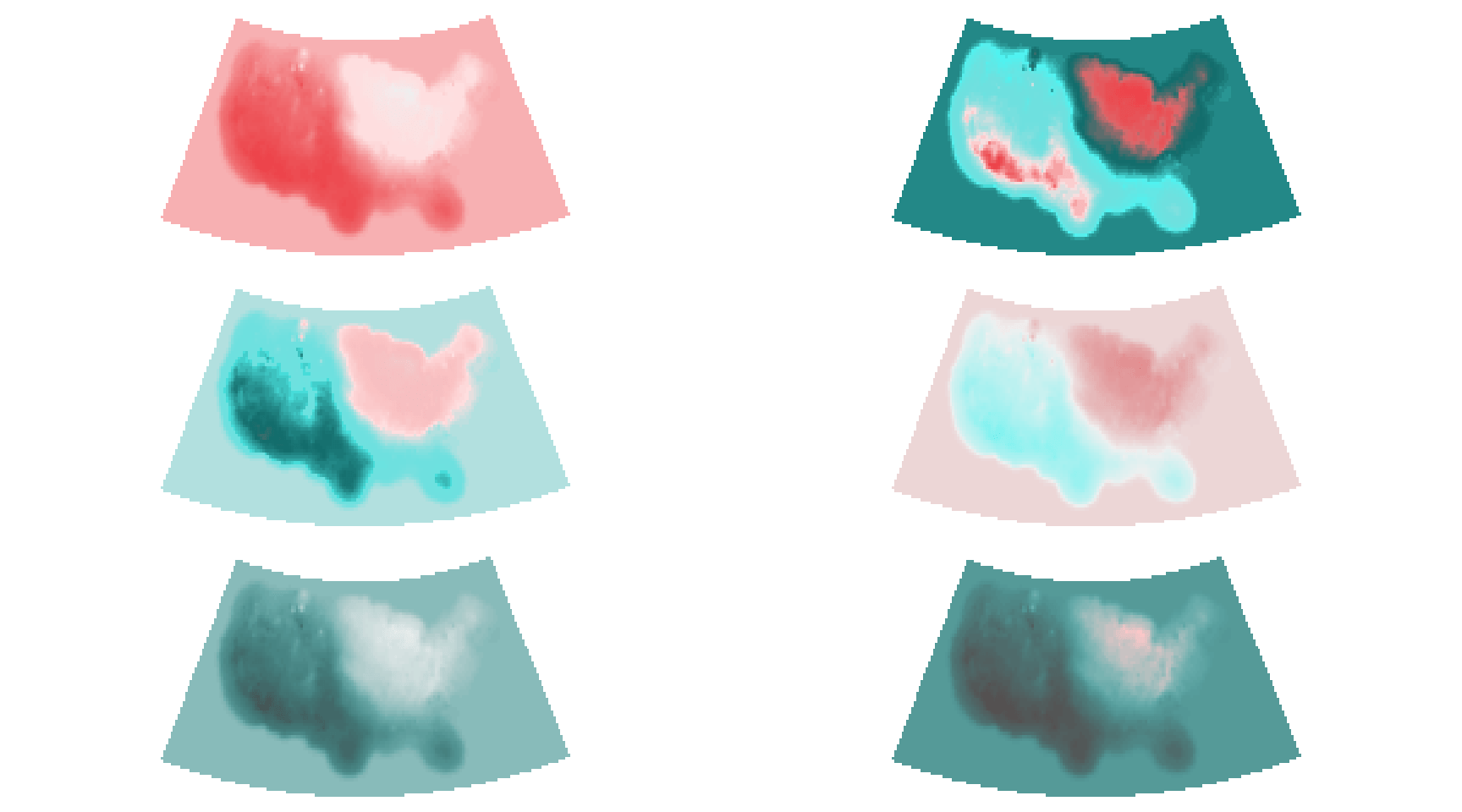# Using the new ‘viridis’ colormap in R (thanks to Simon Garnier)

Nathaniel Smith and Stéfan van der Walt presented a new colormap (for Python) at SciPy 2015 called `viridis`.

From the authors:

The default colourmap in Matplotlib is the colourful rainbow-map called Jet, which is deficient in many ways: small changes in the data sometimes produce large perceptual differences and vice-versa; its lightness gradient is non-monotonic; and, it is not particularly robust against color-blind viewing. Thus, a new default colormap is needed — but no obvious candidate has been found. Here, we present our proposed new default colormap for Matplotlib, and expose the theory, tools, data exploration and motivations behind its design.

You can also find out a tad more about their other colormap designs (a.k.a. the runner-ups), along with Parula, which is a proprietary MATLAB color map.

Simon Garnier (@sjmgarnier) took Nathaniel & Stéfan’s work and turned it into an R package.

Noam Ross (@noamross) & I piled on shortly thereafter to add some ggplot color `scale_` functions which are (for now) only available in Simon’s github repo.

Rather than duplicate the examples already provided in the documentation of those functions, I thought there might be more efficacy in creating a post that helped showcase why you should switch from `rainbow` (et al) to `viridis`.

Since folks seem to like maps, we’ll work with one for the example, but let’s get some package machinations out of the way first:

```library(viridis) library(raster) library(scales) library(dichromat) library(rasterVis) library(httr) library(colorspace)```

Now, we’ll need a map to work with so let’s grab a U.S. max temperature GeoTIFF raster from NOAA (from the bitter cold month of February 2015) and project it to something more reasonable:

```temp_raster <- "http://ftp.cpc.ncep.noaa.gov/GIS/GRADS_GIS/GeoTIFF/TEMP/us_tmax/us.tmax_nohads_ll_20150219_float.tif"   try(GET(temp_raster, write_disk("us.tmax_nohads_ll_20150219_float.tif")), silent=TRUE) us <- raster("us.tmax_nohads_ll_20150219_float.tif")   # albers FTW us <- projectRaster(us, crs="+proj=aea +lat_1=29.5 +lat_2=45.5 +lat_0=37.5 +lon_0=-96 +x_0=0 +y_0=0 +ellps=GRS80 +datum=NAD83 +units=m +no_defs")```

We’ll also make a helper function to save us some typing and setup the base # of colors in the colormap:

```n_col <- 64   img <- function(obj, col) { image(obj, col=col, asp=1, axes=FALSE, xaxs="i", xaxt='n', yaxt='n', ann=FALSE) }```

Let’s take a look at various color palettes with different types of vision. We’ll use a 3×2 grid and:

• use 4 color palettes from `grDevices`,
• make a gradient palette from one of the ColorBrewer sequential palettes, and
• then (finally) use a `viridis` color palette.

We’ll take this grid of 6 maps and view it through the eyes of three different types of color vision as well as a fully desaturated version. Note that I’m not adding much cruft to the map display (including legends) since this isn’t about the values so much as it is about the visual perception of the colormaps.

Remember you can select/click/tap the map grids for (slightly) larger versions.

### “Normal” Vision

```par(mfrow=c(3, 2), mar=rep(0, 4)) img(us, rev(heat.colors(n_col))) img(us, rev(rainbow(n_col))) img(us, rev(topo.colors(n_col))) img(us, rev(cm.colors(n_col))) img(us, gradient_n_pal(brewer_pal("seq")(9))(seq(0, 1, length=n_col))) img(us, rev(viridis(n_col)))```All of the maps convey the differences in max temperature. If you happen to have “normal” color vision you should be drawn to the bottom two (ColorBrewer on the left and Viridis on the right). They are both sequential and convey the temperature changes more precisely (and they aren’t as gosh-awful ugly the the other four).

While the ColorBrewer gradient may be “good”, Viridis is designed to be:

• colorful & “pretty”
• sequential (to not impose other structure on exploratory data analysis visualizations)
• perceptually uniform (i.e. changes in the data should be accurately decoded by our brains) even when desaturated
• accessible to colorblind viewers

and seems to meet those goals quite well.

Take a look at each of the vision-adjusted examples:

### Green-Blind (Deuteranopia)

```par(mfrow=c(3, 2), mar=rep(0, 4)) img(us, dichromat(rev(heat.colors(n_col)), "deutan")) img(us, dichromat(rev(rainbow(n_col)), "deutan")) img(us, dichromat(rev(topo.colors(n_col)), "deutan")) img(us, dichromat(rev(cm.colors(n_col)), "deutan")) img(us, dichromat(gradient_n_pal(brewer_pal("seq")(9))(seq(0, 1, length=n_col)), "deutan")) img(us, dichromat(rev(viridis(n_col)), "deutan"))```### Red-Blind (Protanopia)

```par(mfrow=c(3, 2), mar=rep(0, 4)) img(us, dichromat(rev(heat.colors(n_col)), "protan")) img(us, dichromat(rev(rainbow(n_col)), "protan")) img(us, dichromat(rev(topo.colors(n_col)), "protan")) img(us, dichromat(rev(cm.colors(n_col)), "protan")) img(us, dichromat(gradient_n_pal(brewer_pal("seq")(9))(seq(0, 1, length=n_col)), "protan")) img(us, dichromat(rev(viridis(n_col)), "protan"))```### Blue-Blind (Tritanopia)

```par(mfrow=c(3, 2), mar=rep(0, 4)) img(us, dichromat(rev(heat.colors(n_col)), "tritan")) img(us, dichromat(rev(rainbow(n_col)), "tritan")) img(us, dichromat(rev(topo.colors(n_col)), "tritan")) img(us, dichromat(rev(cm.colors(n_col)), "tritan")) img(us, dichromat(gradient_n_pal(brewer_pal("seq")(9))(seq(0, 1, length=n_col)), "tritan")) img(us, dichromat(rev(viridis(n_col)), "tritan"))```### Desaturated

```par(mfrow=c(3, 2), mar=rep(0, 4)) img(us, desaturate(rev(heat.colors(n_col)))) img(us, desaturate(rev(rainbow(n_col)))) img(us, desaturate(rev(topo.colors(n_col)))) img(us, desaturate(rev(cm.colors(n_col)))) img(us, desaturate(gradient_n_pal(brewer_pal("seq")(9))(seq(0, 1, length=n_col)))) img(us, desaturate(rev(viridis(n_col))))```Hopefully both the ColorBrewer gradient and Viridis palettes stood out as conveying the temperature data with more precision and more consistently across all non-standard vision types as you progressed through each one.

To see this for yourself in your own work, grab Simon’s package and start substituting `viridis` for some of your usual defaults to see if it makes a difference in helping you convey the story your data is trying to tell, both more accurately and for a more diverse audience. Remember, the github version (which will be on CRAN soon) have handy ggplot `scale_` functions to make using `viridis` as painless as possible.

I also updated my Melbourne Walking EDA project to use the viridis palette instead of parula (which I was only really using in defiance of MATLAB’s inane restrictions).Amazon Author Page

## 7 Comments →Using the new ‘viridis’ colormap in R (thanks to Simon Garnier)

1.Henrik Pärn

Thanks for a nice post. However, I believe it is somewhat hard to link each plot to its corresponding line of code. You may consider adding some kind of title/number to the plots and/or a comment in the code. Cheers.

1.hrbrmstr

Thx. It will be clearer in the vignette for the pkg. 0400 post == some shortcuts just to get Simon’s pkg introduced.

2.sbmalev

I was just thinking about how to do this. Excellent timing on this post!

3.Kevin Wright

Interesting, but to my eye these are most decidedly not perceptually uniform. In particular, when I look at a color bar from 0-100 for yellow-green-purple, the yellow part of the band is much too short. There is a big change between 0 (yellow) and 15 (light green), but almost no difference between 43 and 57 (blue-green)

1.hrbrmstr

The science says it is, so you may want to talk to your optometrist.

This site uses Akismet to reduce spam. Learn how your comment data is processed.